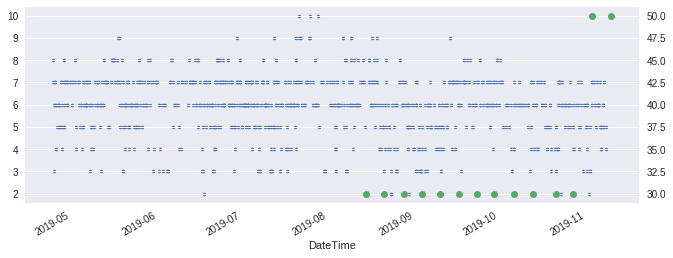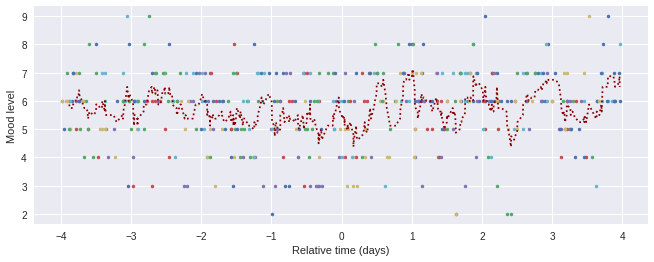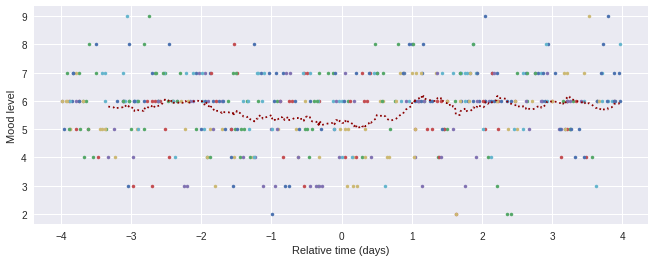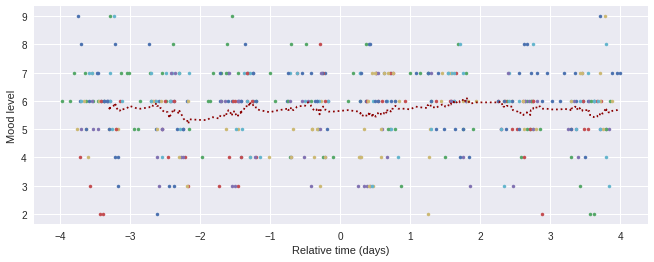# Details for `201911 MPB testosterone and mood (iMoodJournal).ipynb`

#### Description

Analyzes mood data versus specific repeated events (testosterone injections).

Mood is tracked via the iMoodJournal app, exported to CSV, and that file is uploaded to Open Humans. It seems the mood data is very "noisy". My personal result was no clear, dramatic change in mood related to testosterone injections. There might be a shift, but probably needs more data collection to see if this is significant.

#### Tags & Data Sources

iMoodJournal mood testosterone iMoodJournal

### Notebook Last updated 3 weeks, 3 days ago

This notebook uses data exported from iMoodJournal, a mobile app for mood tracking. Is that in your account?

Note from Mad: I created this notebook analyze my mood relative to a series of specific, repeated events: testosterone injections. Testosterone is believed to have an effect on mood; transfolk report low/bad mood associated with lower testosterone before injection, and higher/better moods shortly afterwards. I started testosterone a couple months ago, in August 2019, and I've been tracking my mood using iMoodJournal since May 2019.

## Get packages and load data¶

Information about the code is provided for anyone that's interested.

To start, the code below imports packages this notebook uses.

In :
```from datetime import datetime, timedelta
import io
import os

import arrow
import pandas
import numpy as np
from matplotlib import pyplot
import ohapi
import requests
import seaborn
```

Then, get a list of all available data on Open Humans.

In :
```token = os.environ.get('OH_ACCESS_TOKEN')
user = ohapi.api.exchange_oauth2_member(token)
```

Next, search that data for a datafile that matches the iMoodJournal DataType (id=20).

If this fails, one may need to go back to the top of this notebook and follow instructions for adding this data. Once that's done, it's necessary to run the code above to refresh the file list.

If successful, the file data is loaded into the `raw_data` variable.

In :
```mood_log = [x for x in user['data'] if
'https://www.openhumans.org/api/public/datatype/20/'
in x['datatypes']]
```

Now load this data into a `pandas` data frame. (This notebook is using the `pandas` library for data analysis.)

In :
```datafile = io.StringIO(raw_data.decode('utf-8'))
```

In order to organize and access the data according to timepoints, the code below combines information across columns to create a single index column `DateTime` with the pandas datetime format.

In :
```# Trying to parse different date formats (iMoodJournal export is inconsistent!)...
def get_isoformat(string):
options = ['MMM D, YYYY H m', 'D. MMM YYYY H m']
err = None
for fmt in options:
try:
return arrow.get(string, fmt).isoformat()
except Exception as e:
err = e
continue
raise e

mood['DateTime'] = pandas.to_datetime(
(mood['Date'] + " " + mood['Hour'].map(str) + ' ' + mood['Minute'].map(str)).map(
lambda x: get_isoformat(x)
))
mood = mood.set_index('DateTime')
```

Finally, I'm adding some other personal data I collected: the times I've injected testosterone. This was a copy and paste from my Google spreadsheet.

In :
```injections_raw = """DateTime	Amount (mg)
201908141600	30
201908210500	30
201908280600	30
201909031700	30
201909100600	30
201909162000	30
201909230600	30
201909291200	30
201910060900	30
201910130900	30
201910211500	30
201910271700	30
201911030900	50
201911100900	50"""

datetimes = []
amounts = []
data_in = io.StringIO(injections_raw)
for line in data_in:
data = line.strip().split('\t')
dt = arrow.get(data, 'YYYYMMDDHHmm').isoformat()
datetimes.append(dt)
amounts.append(int(data))

injections = pandas.DataFrame({
'DateTime': pandas.to_datetime(datetimes),
'Amount': amounts,
})
injections = injections.set_index('DateTime')
```

## Graphs!¶

First, let's start with a plot of the full time period to show all mood levels and injections over time.

This isn't very informative, but we can try to see if there's been a general change since starting T.

In :
```seaborn.set(rc={'figure.figsize':(11, 4)})

combined_data = pandas.concat([mood, injections], sort=True)
combined_data['Level'].plot(linewidth=1, style=".")
combined_data['Amount'].plot(style="o", secondary_y=True)
pyplot.show()
```If testosterone is affecting my mood, I expect the strongest "signal" to occur shortly after injection.

Let's calculate and plot mood levels relative to each injection. To do that, I've decided to make a general solution that can be re-used…

Here's a generic function to examine data relative to repeated events.

In :
```def overlay_and_aggregate(df, events, field, before=4, after=4, rolling_avg_n=40):
"""
Overlay and aggregate numeric data from a DateTime indexed dataframe and list of events.

Inputs:
- df: DateTime indexed dataframe
- events: list of DateTimes
- field: field with numeric values in df for overlay and aggregate
- before (default: 7): number of days before each event to graph
- after (default: 7): number of days after each event to graph
- rolling_avg_n (default: 30): number of datapoints to combine for
rolling average across all events
"""
all_rel_times = {}
idx = 0
for event in events:
rel_times = []
amounts = []
for dt in df.index:
diff = arrow.get(dt) - arrow.get(event)
if diff < timedelta(days=after) and diff > timedelta(days=-before):
diff_days = diff.days + diff.seconds/(24 * 60 * 60)
rel_times.append(diff_days)
amounts.append(df[field][dt])
all_rel_times[event.isoformat()] = pandas.DataFrame(
{'Relative time (days)': rel_times, field: amounts}
).set_index('Relative time (days)', drop=False)

combined_data = pandas.DataFrame({'Relative time (days)': [], 'Level': []})
for key in all_rel_times.keys():
all_rel_times[key][field].plot(x='Relative time', style=".")
combined_data = pandas.concat([combined_data, all_rel_times[key]], sort=True)

combined_data = combined_data.sort_values('Relative time (days)')
combined_data['average'] = combined_data.iloc[:,0].rolling(window=rolling_avg_n).mean()
plot = combined_data['average'].plot(style=':', color='darkred')
return plot
```

### Graph mood vs. injection times¶

My first effort used an averaging window of 8. This seemed very noisy.

In :
```plot = overlay_and_aggregate(mood, injections.index, 'Level', rolling_avg_n=8)
plot = plot.set_ylabel("Mood level")
```But increasing the window size to 40: I do seem to see a mood increase in the day after injection?

In :
```plot = overlay_and_aggregate(mood, injections.index, 'Level', rolling_avg_n=40)
plot = plot.set_ylabel("Mood level")
```### Is this just a weekly rhythm?¶

My injections are weekly, and recently they occur on Sundays. What if I simply have lower moods on the weekends – might that explain this pattern?

One way to check is to shift my injections around the week and continue collecting data.

Another is to check if I see the same pattern if I map moods against the start of each week – I can re-use my function to do this right away, by mapping against the start of Monday each week.

In :
```start_date = min(injections.index)
end_date = max(mood.index)
first_monday = arrow.get(start_date).floor("week").shift(days=1).floor("day")

week_starts = [a.datetime for a in arrow.Arrow.range("week", first_monday, end_date)]

plot = overlay_and_aggregate(mood, week_starts, 'Level', rolling_avg_n=40)
plot = plot.set_ylabel("Mood level")
```## Conclusions¶

I'm leaning towards believing there really is a shift!

Initially I thought "no pattern": I'm very skeptical and cautious of making inferences from data, my experience in research has taught me there are many confounders and false leads.

But with a sufficiently large averaging window, there seems to be a genuine change to the average data. Also, the one confounder I expected – a weekly pattern – doesn't seem to explain the pattern.

If testosterone is affecting my mood, I expect the strongest "signal" to occur shortly after injection.

### Thank you¶

Many thanks to Eric Jain and Bastian Greshake Tzovaras, whose feedback was brief but sufficient to nudge me to revisit the analysis (twice now!) to add (a) a larger averaging window, and (b) check the weekly patterns.